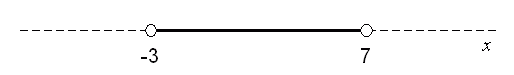Subject: algebra two Name: Alyssa Who are you: Student 2x > -6 and x - 4 < 3 , i need to know how to solve it and how to graph it. Hi Alyssa. First, "solve" for x in both inequalities: 2x > -6 is the same as -3 < x. and x - 4 < 3 is the same as x < 7 The situation is that both of these statements are true at the same time, so -3 < x < 7. In other words, x has a value between -3 and 7, but not including exactly -3 or 7. To "graph" this, you would use a number line and mark -3 to the left of 7 and draw circles that are not filled in (to indicate that exactly -3 and 7 are not included), and thicken the line between the circles (to indicate this range is the solution). Here's how I would draw it:Stephen La Rocque.>